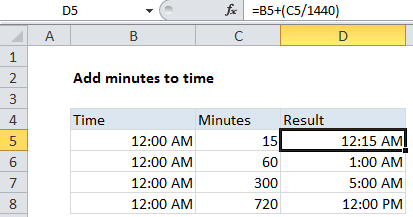## Excel Office

Excel How Tos, Tutorials, Tips & Tricks, Shortcuts

# Add decimal minutes to time in Excel

It you need to add decimal minutes to time in Excel then this tutorials is for you. See example below:

To add a given number of minutes to a time, you can add minutes divided by 1440, or use the TIME function.

## Formula

`=time+(minutes/1440)`## Explanation

In the example shown, the formula in D5 is:

`=B5+(C5/1440)`

Note: make sure results are formatted as time.

### How this formula works

Times in Excel are factional values of 24 hours. One hour of time is 1/24, and 1 minute of time is 1/(24*60) = 1/1440.

Also See:   Sum every nth column in Excel

As a result, if you have a decimal value for 15 minutes, and a time in A1, you can add 15 minutes of time to the value in A1 like this:

`=A1+(15/1440)`

### With the TIME function

You can also add time values with the TIME function. To add 15 minutes to a time in A1, use:

`=A1+TIME(0,15,0)`

The TIME function saves you from having to remember the formula for converting decimal minutes to an Excel time. However, note that the TIME function will “roll over” back to zero when values exceed 24 hours.

Also See:   Excel Get number at place value Example

For example, 25 hours of time is 1500 minutes. Note the differences:

```=TIME(0,1500,0) = 0.041667 = 1:00 AM same day
=1500/1440 = 1.041667 = 1:00 AM next day```

### Subtracting minutes from time

You may get an error if you try to subtract minutes from a time, when the result is negative, because Excel doesn’t allow negative time values.

Also See:   Excel Data validation number multiple 100

One way to avoid this problem is to use a formula like this:

`=MOD(time-(minutes/1440),1)`

Here MOD function takes care of the negative problem by using the MOD function to “flip” negative values to the required positive value.